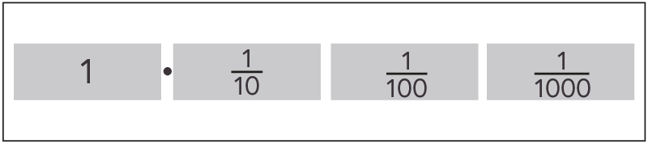## Want to keep learning?

This content is taken from the National STEM Learning Centre's online course, Maths Subject Knowledge: Fractions, Decimals, and Percentages. Join the course to learn more.
2.6

## National STEM Learning Centre

Skip to 0 minutes and 7 seconds MICHAEL ANDERSON: So let’s have a look at converting decimals into fractions. We’re going to start with decimals that terminate. What’s really important with these examples is the place value of the last digit. So let’s have a look at 0.3. Now, here, the last digit is the 3. And that’s in the tens column. So to convert this into a fraction is dead easy. This is just 3/10, so 0.3 is 3/10. Now, if we look at another fraction, 0.03, here we have a 0 in the tens column and a 3 in the hundreds column. We can see that this is 3 hundredths. So this is going to be equal to 3/100. OK?

Skip to 0 minutes and 50 seconds So the next example, let’s have a look at something like 0.12. Now, the final digit in this decimal is this 2. And that’s in the hundreds column. So this is 1, 2, 12/100. But this isn’t our final answer because 12/100 can be simplified. Both of them are even numbers, so I can divide both by 2 to get 6/50. Both of these are even numbers, so I can divide them both again to get 3/25. So in its simplest form, 0.12 is 3/25. The last example that I wanted to talk about was 0.125. If you’d like to have a go at this question yourself, pause the video and then resume when you’d like to see the answer.

Skip to 1 minute and 40 seconds So with the 0.125 example, the final digit is actually in the thousands column. So we’re going to write this as a fraction as 1 2 5, 125/1,000. And now the tricky bit is making sure that it’s in its simplest form. Now, 125 and 1000 are both divisible by 5. So if I divide them both by 5, I get 25/200. Now, these are both divisible by, well, actually, 5, but 25 I’ve just spotted. So 25 goes into 25 once. And 25 into 200, that goes 8 times. So 1/8 is the same as 0.125 as a decimal.

# Decimals as fractions: terminating decimals

In the last few steps we saw that some fractions, when expressed as a decimal, are terminating decimals in that they have a finite number of decimal places.

## What is 0.125 expressed as a fraction?

In this video, Michael and Paula consider how to express a terminating decimal as a fraction. You’ll see that they use the place value of the last digit of the decimal to produce a fraction.However, where possible, that fraction is simplified. What we mean by this, is that we find the equivalent fraction where the denominator has the smallest number. We look at simplification in more detail in step 3.7 next week.

## Problem worksheet

Now complete question 3 from this week’s worksheet.

Just a reminder, each week’s worksheet can be downloaded from the first step of the week.